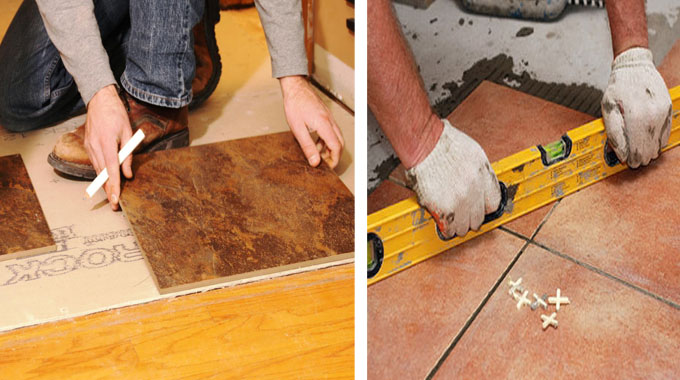# How to work out the quantity of tiles with different shapesThis civil engineering tutorial will teach you how to work out the tiles in the floor of a room of any apartment. In order to make calculation, just find the area of filing floor. Obtain the dimension of two opposite direction and multiply them.

So, the quantity of floor tiles = Length x Width

### The following two methods are commonly utilized to calculate tiles :-

Average Method
Counting Method

Under this method, obtain the area of the floor, the skirting area as well as add wastage. The area of the floor can be determined from the drawing or by taking measurement practically.

For obtaining the skirting area, initially, find out the total length of the skirting and multiply the length with the height of the skirting. After that, add the area of skirting with the floor area.

For wastage, 10% extra can be added with the actual tile quantity.

### So, the quantity of floor tiles should be calculated with the following formula :-

Floor tiles area + Skirting area + 10% extra

Counting Method

Under this method, it is required to generate a shop drawing in AutoCAD. Now, open the floor plan in AutoCAD and draw lines on the plan. Once the shop drawing is created, take a print out. Now, count the number of tiles necessary for the floor from the drawing. Also count the number of tiles for skirting.

Once the total numbers of tiles are found, multiply the number of tiles with the size of a tile to determine the quantity.

Under this method, no wastage should be taken. But, in case of retaining some tiles for future repairing purpose, consider 3% wastage.

### So, the quantity of floor tiles will be calculated as follow :-

Number of tiles x Size of tiles + 3% wastage

Calculation of area of tiles with different shapes (circular, trapezoidal, etc)

### Area :-

• For square floor = 2? length.
• For rectangular floor = Length ? Width.
• For a circular floor = pr2 (p=3.14, r=radius).
• For a trapezium shaped floor = {(a+b)?2} ? h (a=longer base, b=shorter base, h=height).